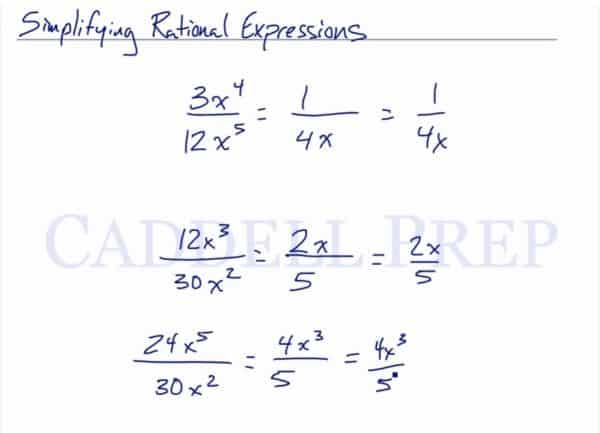In this video, we are going to simplify rational expressions. After you finish this lesson, view all of our Algebra 1 lessons and practice problems.

For example:$\dfrac{3x^4}{12x^5}$
It’s just like reducing fractions. Begin by simplifying the constants.$\dfrac{3}{12}$ would be$\dfrac{1}{4}$
Let’s now look at the exponents. The numerator is to the fourth power while the denominator is to the fifth power. As a result, there will be one x in the denominator but not in the numerator.$\dfrac{1x^4}{4x^5}$$\dfrac{1}{4x}$

Now let’s look at an example where there is an operation taking place in the numerator.$\dfrac{24x^5-15x^3}{6x^4}$
Here we can separate into two separate fractions$\dfrac{24x^5}{6x^4}$ and$\dfrac{-15x^3}{6x^4}$
Then we can simplify each one and get a final answer of$6x-\dfrac{-5}{2x}$

When there is an operation in the denominator, we cannot simply do the same thing. Instead, we must factor each term by its greatest common factor. For example:$\dfrac{6x^4}{24x^5-15x^3}$
The greatest common factor for all three terms is$3x^3$. So if we take out a$3x^3$ from each term, we are left with$\dfrac{3x^3(2x)}{3x^3(8x^2-5)}$
Then, the$3x^3$ in the numerator and denominator will cancel out, leaving us with a final answer of$\dfrac{2x}{8x^2-5}$

Another type of rational expression may look like$\dfrac{x^2-x-12}{x^2-2x-8}$
To solve something like this, we can factor the quadratic in the numerator and denominator. In this example, we would have$\dfrac{(x-4)(x+3)}{(x-4)(x+2)}$
From here, we could cancel out the (x-4) from the numerator and denominator, leaving us with$\dfrac{x+3}{x+2}$

Examples of Simplifying Rational Expressions

Example 1

Simplify$\dfrac{x^2-9x-14}{x^2+2x-8}$

First, factor both the numerator and denominator of the fraction.$\dfrac{(x-2)(x-7)}{(x-2)(x+4)}$
Then, reduce the fraction by cancelling out$(x-2)$$\dfrac{(x-7)}{(x+4)}$

Example 2

Simplify$\dfrac{x^2+x-12}{x^2+10x+24}$

First, factor both the numerator and denominator of the fraction.$\dfrac{(x+4)(x-3)}{(x+4)(x+6)}$
Then, reduce the fraction by cancelling out$(x+4)$$\dfrac{(x-3)}{(x+6)}$

Video-Lesson Transcript

Let’s go over how to simplify rational expressions.

For example, we have$\dfrac{3x^4}{12x^5}$

We want to reduce this.

What we have to do is reduce it just like reducing normal fractions.

Reduce the constant by finding the greatest common factor. Which is$3$.

Then, to divide the$x$.

The$x$-term stays at the denominator because$5$ is greater than$4$.$= \dfrac{1}{4x}$

Let’s try this one$\dfrac{12x^3}{30x^2}$$6$ goes into$12$ and$30$.

When dividing the$x$, let’s subtract$2$ from$3$.$= \dfrac{2x}{5}$

Let’s look at one more$\dfrac{24x^5}{30x^2}$

Here, think of a number that goes into both of these.$6$ does. Then divide$24$ and$30$.

Then subtract the exponents of$x$.$= \dfrac{4x^3}{5}$The difference on the exponent stays at the numerator because it’s higher.

Now, let’s look at these two examples.$\dfrac{24x^5 - 15x^3}{6x^4}$

This one has a binomial in the numerator and a monomial in the denominator.

If we have two terms separated by a plus or a minus sign, we can actually split them up.

We can write this down as$= \dfrac{24x^5}{6x^4} - \dfrac{15x^3}{6x^4}$

Then let’s solve each$= \dfrac{6x}{1} - \dfrac{5}{2x}$$= 6x - \dfrac{5}{2x}$

Let’s look at another one.$\dfrac{6x^4}{24x^5 - 15x^3}$

It looks like the first example but it’s flipped. This has a monomial numerator and a binomial denominator.

In the first example, we can split the binomial into two because it’s in the numerator.

Here, we cannot.

So, we keep it as it is. And then reduce it from here.$\dfrac{6x^4}{24x^5 - 15x^3}$

Now, let’s get the greatest common factor which goes into all of these terms.

What number goes into$6$,$24$, and$15$?

Well,$3$ does.

Then let’s get the lowest exponent for$x$.

Now, let’s factor$=\dfrac{3x^3 (2x)}{3x^3 (8x^2 - 5)}$$=\dfrac{2x}{8x^2 - 5}$

The two examples look similar but we end up with different answers.

This is another type of rational expression.$\dfrac{x^2 - x - 12}{x^2 - 2x - 8}$

Since the numerator is separated by minus, we can split them up.$= \dfrac{x^2}{x^2 - 2x - 8} - \dfrac{x}{x^2 - 2x - 8} - \dfrac{12}{x^2 - 2x - 8}$

This is how to try to do it.

We can’t get very far in reducing this. There is only one greatest common factor for each.

In this case, this is not the best method.

What we want to do is just to factor from the beginning.$\dfrac{x^2 - x - 12}{x^2 - 2x - 8}$$= \dfrac{(x - 4) (x + 3)}{(x - 4) (x + 2)}$

Now that we have two factors, we can cancel this out.

And we’re left with$= \dfrac{x + 3}{x + 2}$